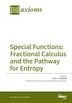Special Functions: Fractional Calculus and the Pathway for Entropy

Guest editorHaubold J. Hans

Pricing and Purchase Info

\$105.80

Earn 529 plum® points

Prices and offers may vary in store

Quantity:

In stock online

Ships free on orders over \$25

Not available in stores

Historically, the notion of entropy emerged in conceptually very distinct contexts. This book deals with the connection between entropy, probability, and fractional dynamics as they appeared, for example, in solar neutrino astrophysics since the 1970's (Mathai and Rathie 1975, Mathai and Pederzoli 1977, Mathai and Saxena 1978, Mathai, Saxena, and Haubold 2010).

The original solar neutrino problem, experimentally and theoretically, was resolved through the discovery of neutrino oscillations and was recently enriched by neutrino entanglement entropy. To reconsider possible new physics of solar neutrinos, diffusion entropy analysis, utilizing Boltzmann entropy, and standard deviation analysis was undertaken with Super-Kamiokande solar neutrino data. This analysis revealed a non-Gaussian signal with harmonic content. The Hurst exponent is different from the scaling exponent of the probability density function and both Hurst exponent and scaling exponent of the Super-Kamiokande data deviate considerably from the value of ½, which indicates that the statistics of the underlying phenomenon is anomalous. Here experiment may provide guidance about the generalization of theory of Boltzmann statistical mechanics.

Arguments in the so-called Boltzmann-Planck-Einstein discussion related to Planck's discovery of the black-body radiation law are recapitulated mathematically and statistically and emphasize from this discussion is pursued that a meaningful implementation of the complex ‘entropy-probability-dynamics’ may offer two ways for explaining the results of diffusion entropy analysis and standard deviation

analysis. One way is to consider an anomalous diffusion process that needs to use the fractional space-time diffusion equation (Gorenflo and Mainardi) and the other way is to consider a generalized Boltzmann entropy by assuming a power law probability density function. Here new mathematical framework, invented by sheer thought, may provide guidance for the generalization of Boltzmann statistical mechanics.

In this book Boltzmann entropy, generalized by Tsallis and Mathai, is considered. The second one contains a varying parameter that is used to construct an entropic pathway covering generalized type-1 beta, type-2 beta, and gamma families of densities. Similarly, pathways for respective distributions and differential equations can be developed. Mathai's entropy is optimized under various conditions reproducing the well-known Boltzmann distribution, Raleigh distribution, and other distributions used in physics. Properties of the entropy measure for the generalized entropy are examined. In this process the role of special functions of mathematical physics, particularly the H-function, is highlighted.

Title:Special Functions: Fractional Calculus and the Pathway for EntropyFormat:PaperbackProduct dimensions:304 pages, 9.61 × 6.69 × 0.83 inShipping dimensions:9.61 × 6.69 × 0.83 inPublished:January 17, 2018Publisher:MDPI AGLanguage:English

The following ISBNs are associated with this title:

ISBN - 10:3038426652

ISBN - 13:9783038426653